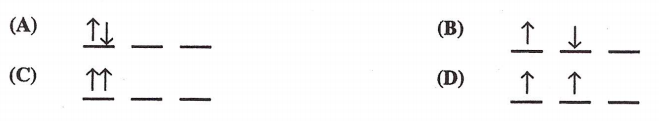Chemistry Practice Problems Orbital Diagrams Practice Problems Solution: Which of these electron diagrams could represent t...

🤓 Based on our data, we think this question is relevant for Professor Prado's class at AU.

# Solution: Which of these electron diagrams could represent the ground state of the p valence electrons of carbon?

###### Problem

Which of these electron diagrams could represent the ground state of the p valence electrons of carbon?Orbital Diagrams

Orbital Diagrams

#### Q. How many orbitals are there in the third shell ( n=3)? Express your answer numerically as an integer. Show the orbital-filling diagram for N (nitrogen...

Solved • Fri Dec 15 2017 14:25:48 GMT-0500 (EST)

Orbital Diagrams

#### Q. What is the electron configuration for Carbon? What is the orbital diagram for carbon?

Solved • Fri Dec 15 2017 13:54:48 GMT-0500 (EST)

Orbital Diagrams

#### Q. Fill in the energy orbital Diagram for the following atom Cr(III). Be sure to label orbitals by n and l quantum numbers, and place electrons in the ap...

Solved • Fri Apr 22 2016 10:51:58 GMT-0400 (EDT)

Orbital Diagrams

#### Q. Choose the orbital diagram that represents the ground state of N.

Solved • Fri Mar 11 2016 12:54:46 GMT-0500 (EST)